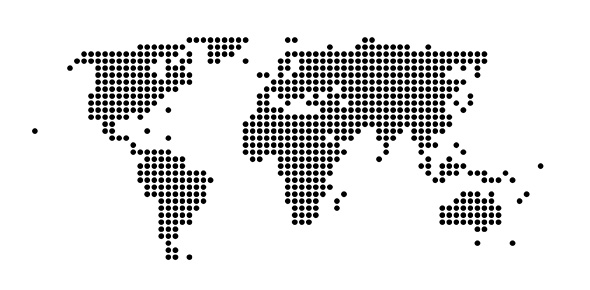# 6:6:1 Scale Drawings

3 QuestionsSettingsRelated Topics
• 1.
Round answers to the nearest hundredth if necessary.The scale factor for a house is 2.5 inches = 14 feet.  What are the dimensions of a room if the blueprint is 3 inches by 5 inches.  (HINT:  There will be 2 answers).
• A.

6 ft by 7 ft

• B.

9 ft by 12 ft

• C.

13 ft by 15 ft

• D.

16.8 ft by 28 ft

• 2.
Round answers to the nearest hundredth if necessary.The scale factor for a model is 8 in = 125 cm.  Find the actual distance if the distance on the model is 244 cm.
• A.

15.62 in.

• B.

122 in.

• C.

75 in.

• D.

53.87 in.

• 3.
Round answers to the nearest hundredth if necessary.The scale of a map is 2 cm = 3.6 miles.  How many miles are represented on a map by 9 cm?
• A.

8.1 miles

• B.

12.8 miles

• C.

16.2 miles

• D.

25.6 miles